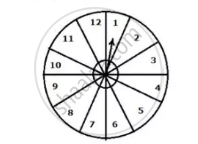Share

# A Game Consists of Spinning Arrow Which Ccomes to Rest Pointing at One of the Number 1,2,3,4,5,6,7,8,9,10,11,12; as Shown Below - Mathematics

Course

#### Question

A game consists of spinning arrow which ccomes to rest pointing at one of the number 1,2,3,4,5,6,7,8,9,10,11,12; as shown belowIf the outcomes are equally likely, find the probability that the pointer will point at :

(1) 6

(2) an even number

(3) a prime number

(4) a number greater than 8

(5) a number   less than or equal to 9

(6) a number between 3 and 11

#### Solution

Total number of possible outcomes =12

(1) Number of favorable outcomes for 6=1

P(the pointer will point a 6) = 1/12

(2)favorable outcomes for an even number are 2,4,6,8,10,12

Number of favorable outcomes=6

P( the pointer will be at an even number)=6/12=1/2

(3) Favorable outcomes for a prime number are 2,3,5,7,11

Number of favorable outcomes=5

p(the pointer will be at a prime number)=5/12

(4) Favourable outcomes for a number greater than 8 are 9,10,11,12

Number of favorable  outcomes=4

P(the pointer will be at a number greater than 8 )=4/12=1/3

(5) Favorable outcomes for a number less than or equal  to are 1,2,,3,4,5,6,7,8,9

Number of favorable outcome =9

p(the pointer will be at a number less than or equal to 9)= 9/12=3/4

(6) Favorable outcomes for a number between 3 and 11 are 4,5,6,7,8,9,10

Number of favorable outcomes=7

P(the pointer will be at a number 3 and 11) =7/12

Is there an error in this question or solution?